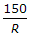# Civil Engineering - Highway Engineering

### Exercise :: Highway Engineering - Section 4

1.

Round-abouts are not suitable if number of vehicles exceed

 A. 3000 B. 4000 C. 5000 D. 6000

Explanation:

No answer description available for this question. Let us discuss.

2.

For a comfortable travel on Highways, the centrifugal ratio should not exceed

 A. 0.1 B. 0.15 C. 0.2 D. 0.25 E. 0.3

Explanation:

No answer description available for this question. Let us discuss.

3.

If V is speed in km/hour and R is radius of the curve, the superelevation e is equal to

 A.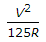B.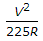C.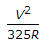D.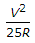Explanation:

No answer description available for this question. Let us discuss.

4.

The traffic manoeuvre means

 A. diverging B. merging C. crossing D. all the above.

Explanation:

No answer description available for this question. Let us discuss.

5.

To compensate the loss of tractive force of vehicles along curves of radius R, the percentage reduction of gradient, is

 A.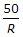B.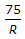C.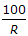D.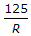E.